## NCERT Exemplar Solutions for Class 10 Science Chapter 1 Chemical Reactions and Equations

These Solutions are part of NCERT Exemplar Solutions for Class 10 Science. Here we have given NCERT Exemplar Solutions for Class 10 Science Chapter 1 Chemical Reactions and Equations

NCERT Exemplar Solutions for Class 10 Science Chapter 1 Multiple Choice Questions

Question 1.
Which of the following is not a physical change ?
(a) Boiling of water to give water vapours
(b) Melting of ice to give water
(c) Dissolution of salt in water
(d) Combustion of Liquefied Petroleum Gas (LPG)
(d). LPG is a mixture of gases like propane (C3Hg) and butane (C4H10) in liquefied form. These are chemical substances and undergo combustion upon heating with air or oxygen. It is therefore, a chemical change.

More Resources

Question 2.
The following reaction is an example of a
4NH3(g) + SO2(g) ————-> 4NO(g) + 6H2O(g)
(i) displacement reaction
(ii) combustion reaction
(iii) redox reaction
(iv) neutralisation reaction
(a) (i) and (iv)
(b) (ii) and (iii)
(c) (i) and (ii)
(d) (iii) and (iv)
(c). Oxygen has displaced hydrogen from NH3. It is also a combustion reaction.

Question 3.
Which of the following statements about the given reaction are correct ?
3Fe(s) + 4H2O(g) ———-> Fe3O4(s) + 4H2(g)
(i) Iron metal is getting oxidised
(ii) Water is getting reduced
(iii) Water is acting as reducing agent
(iv) Water is acting as oxidising agent
(a) (i), (ii) and (iii)
(b) (iii) and (iv)
(c) (i), (ii) and (iv)
(d) (ii) and (iv).
(c). Statements (i), (ii) and (iv) are all correct.

Question 4.
Which of the following are exothermic processes ?
(i) Reaction of water with quick lime
(ii) Dilution of an acid
(iii) Evaporation of water
(iv) Sublimation of camphor (crystals)
(a) (i) and (ii)
(b) (ii) and (iii)
(c) (i) and (iv)
(d) (iii) and (iv)
(a).
(i) Reaction of quick lime with ; water and (ii) dilution of an acid are both exothermic processes. The other two processes (iii) and (iv) are of endothermic nature.

Question 5.
Three beakers labelled as A, B and C each containing 25 mL of water were taken. A small amount of NaOH, anhydrous CuS04 and NaCl were added to the beakers A, B and C respectively. It was observed that there was an increase in the temperature of the solutions contained in beakers A and B, whereas in case of beaker C, the temperature of the solution fell. Which one of the following statement(s) is(are) correct ?
(i) In beakers A and B, exothermic process has occurred.
(ii) In beakers A and B, endothermic process has occurred.
(iii) In beaker C exothermic process has occurred.
(iv) In beaker C endothermic process has occurred.
(a) (i) only
(b) (ii) only
(c) (i) and (iv)
(d) (ii) and (iii)
(c).

Question 6.
A dilute ferrous sulphate solution is gradually added to the beaker containing acidified permanganate solution. The light purple colour of the solution fades and finally disappears. Which of the following is the correct explanation for the observation ?
(a) KMnO4 is an oxidising agent and it oxidises FeSO4
(b) FeSO4 acts as an oxidising agent and it oxidises KMnO4
(c) The colour disappears due to dilution; no reaction is involved
(d) KMnO4 is an unstable compound and decomposes in presence of FeSO4 to a colourless compound.
(a). Potassium permanganate (KMnO4) is an oxidising agent. It oxidises ferrous sulphate to ferric sulphate in the presence of dilute
H2SO4.By taking part in the reaction, the light purple colour of the solution slowly fades and finally disappears.

Question 7.
Which among the following is(are) double displacement reaction(s) ?
(i) Pb + CuCl2 ————> PbCl2 + Cu
(ii) Na2SO4 + BaCl2 ———-> BaSO4 + 2NaCl
(iii) C + O ———–> CO2
(iv) CH4 + 2O ———> CO2 + 2H2O
(a) (i) and (iv)
(b) (ii) only
(c) (i) and (ii)
(d) (iii) and (iv)
(b). Only reaction (ii) is double displacement in nature.

Question 8.
Which among the following statement(s) is(are) true ? ‘Exposure of silver chloride to sunlight for a long duration turns grey due to
(i) the formation of silver by decomposition of silver chloride
(ii) sublimation of silver chloride
(iii) decomposition of chlorine gas from silver chloride
(iv) oxidation of silver chloride
(a) (i) only
(b) (i) and (iii)
(c) (ii) and (iii)
(d) (iv) only
(a). Silver chloride is sensitive to sun light and decomposes slowly to give silver which is grey in colour and chlorine.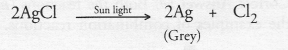Question 9.
Solid calcium oxide reacts vigorously with water to form calcium hydroxide accompanied by liberation of heat. This process is called slaking of lime. Calcium hydroxide dissolves in water to form a solution called lime water. Which among the following is (are) true about slaking of lime and the solution formed ?
(i) It is an endothermic reaction
(ii) It is an exothermic reaction
(iii) The pH of the resulting solution will be more than seven
(iv) The pH of the resulting solution will be less than seven
(a) (i) and (ii)
(b) (ii) and (iii)
(c) (i) and (iv)
(d) (iii) and (iv).
(b). Slaking of lime is an exothermic process. Lime (CaO) dissolves in water to form calcium hydroxide called slaked lime Ca(OH)2. Since it is a base, the pH of solution will become more than seven (7).

Question 10.
Barium chloride on reacting with ammonium sulphate forms barium sulphate and ammonium chloride. Which of the following correctly represents the type of the reaction involved ?
(i) Displacement reaction
(ii) Precipitation reaction
(iii) Combination reaction
(iv) Double displacement reaction
(a) (i) only
(b) (ii) only
(c) (iv) only
(d) (ii) and (iv).
(d). A white precipitate of BaSO4 is formed. The reaction is double displacement in nature.

Question 11.
Electrolysis of water is a decomposition reaction. The mole ratio of hydrogen and oxygen gases liberated during electrolysis of water is
(a) 1:1
(b) 2:1
(c) 4:1
(d) 1:2.
(b).

Question 12.
Which of the following is(are) an endothermic process (es) ?
(i) Dilution of sulphuric acid
(ii) Sublimation of dry ice
(iii) Condensation of water vapours
(iv) Evaporation of water
(a) (i) and (iii)
(b) (ii) only
(c) (iii) only
(d) (ii) and (iv)
(d). Both sublimation of dry ice (ii) and evaporation of water (iv) are of endothermic nature.

Question 13.
In the double displacement reaction between aqueous potassium iodide .and aqueous lead nitrate, a yellow precipitate of lead iodide is formed. While performing the activity if lead nitrate is not available, which of the following can be used in place of lead nitrate ?
(c) Ammonium nitrate
(d) Potassium sulphate.
(b). Lead sulphate being insoluble in water will not react. However, lead acetate can be used since it is water soluble in nature.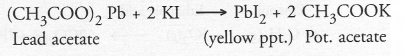Question 14.
Which of the following gases can be used for storage of fresh sample of an oil for a long time ?
(a) Carbon dioxide or oxygen
(b) Nitrogen or oxygen
(c) Carbon dioxide or helium
(d) Helium or nitrogen.
(d). Both helium (He) and nitrogen (N2) can be used. In their presence, rancidity of fresh sample of an oil will not take place.

Question 15.
The following reaction is used for the preparation of oxygen gas in the laboratory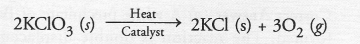Which of the following statement(s) is(are) correct about the reaction ?
(a) It is a decomposition reaction and is endothermic in nature
(b) It is a combination reaction
(c) It is a decomposition reaction and is accompanied by release of heat
(d) It is a photochemical decomposition reaction and exothermic in nature
(a).

Question 16.
Which one of the following processes involve chemical reactions ?
(a) Storing of oxygen gas under pressure in a gas cylinder
(b) Liquefaction of air
(c) Keeping petrol in a china dish in the open
(d) Heating copper wire in the presence of air at high temperature.
(d). Copper will react with oxygen present in air upon strong heating to form copper (II) oxide or cupric oxide.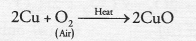Question 17.
In which of the following chemical equations, the abbreviations represent the correct states of the reactants and products involved at reaction temperature ?
(a) H2(l) + O2(l) ———–> 2H2O(g)
(b) H2(g) + O2(l) ———>2H2O(l)
(c) 2H2(g) + O2(g) ———> 2H2O(l)
(d) 2H2(0) + O2(g) ——–> 2H2O(g)
(d). The reaction is carried upon strong heating. At this temperature, H2O formed is in gaseous state.

Question 18.
Which of the following are combination reactions ?
(i)  2KClO3  ———-> 2KCl + 3O
(ii) MgO + H2O ———–> Mg(OH)2
(iii) 4Al + 3O2 ———–> 2Al2O3
(iv) Zn + FeSO———-> ZnSO4 + Fe
(a) (i) and (iii)
(b) (iii) and (iv)
(c) (ii) and (iv)
(d) (ii) and (iii).
(d). Both the reactions (ii) and (iii) are the examples of combination reactions.

NCERT Exemplar Solutions for Class 10 Science Chapter 1 Short Answer Questions

Question 19.
Write the balanced chemical equations for the following reactions and identify the type of reaction in each
(a) Nitrogen gas is treated with hydrogen gas in the presence of a catalyst at 773 K to form ammonia gas.
(b) Potassium hydroxide solution is treated with acetic acid to form potassium acetate and water.
(c) Ethanol is warmed with ethanoic acid to form ethyl acetate in the presence of concentrated H2SO4.
(d) Ethene is burnt in the presence of oxygen to form carbon dioxide, water and to release heat and light. Catalyst (Pt)Question 20.
Write the balanced chemical equations for the following reactions and identify the type of reaction in each case.
(a) Iron (III) oxide reacts with aluminium and gives molten iron and aluminium oxide. (CBSE 2012)
(b) Magnesium ribbon is burnt in an atmosphere of nitrogen gas to form solid magnesium nitride.
(c) Chlorine gas is passed in an aqueous potassium iodide solution to form potassium chloride solution and solid iodine.
(d) Ethanol is burnt in air to form carbon dioxide, water and releases heat. (CBSE 2013)Question 21.
Complete the missing components/variables given as x and y in the following reactions
(a) Pb(NO3)2 (aq) + 2KI(aq) ———>PbI2(x) + 2KNO3(y)
(b) Cu(s) + 2AgNO3(aq) ————> Cu(NO3)2(aq) + x(s)
(c) Zn(s) + H2SO4(aq) ———–> ZnSO4(x) + H2(g)
(d) CaCO3(s)  ————>  CaO(s) + CO2(g)
(a) x = yellow precipitate, y = (aq)
(b) x = 2Ag(s)
(c) x = (aq) ; y = (g)
(d) x = heat.

Question 22.
Which among the following changes are exothermic or endothermic in nature ?
(a) Decomposition of ferrous sulphate
(b) Dilution of sulphuric acid
(c) Dissolution of sodium hydroxide in water
(d) Dissolution of ammonium chloride in water
(a) Endothermic
(b) Exothermic
(c) Exothermic
(d) Endothermic.

Question 23.
Identify the reducing agent in the following reactions
Ammonia (NH3) is the reducing agent. It has reduced oxygen (O2) which acts as oxidising agent.
(b) Water (H2O) is the reducing agent. It has reduced fluorine (F2) which acts as oxidising agent.
(c) Carbon monoxide (CO) is the reducing agent. It has reduced ferric oxide (Fe2O3) which acts as oxidising agent.
(d) Hydrogen (H2) is the reducing agent. It has reduced oxygen (O2) which acts as oxidising agent.

Question 24.
Identify the oxidising agent (oxidant) in the following reactions
(a) Pb3O4 + 8HCl ———-> 3PbCl2 + Cl2 + 4H2O
(b) 2Ca + O———-> 2CaO
(c) CuSO4 + Zn ———-> Cu + ZnSO4
(d) V2O5 + 5Ca ————> 2V + 5Ca
(e) 3Fe + 4H2O ———–> Fe3O4 + 4H2
(f) ZnO + H2 ———–> Zn + H2O
(a) Pb3O4
(b) O2
(c) CuSO4
(d) V2O5
(e) H2O
(f) ZnO

Question 25.
Write the balanced chemical equations for the following reactions
(a) Sodium carbonate on reaction with hydrochloric acid in equal molar concentrations gives sodium chloride and sodium hydrogen carbonate.
(b) Sodium hydrogen carbonate on reaction with hydrochloric acid gives sodium chloride, water and liberates carbon dioxide.
(c) Copper sulphate on treatment with potassium iodide precipitates cuprous iodide (Cu2I2), liberates iodine gas and also forms potassium sulphate.
Identify the reducing agent in the following reactions
(a) Na2CO3 (s) + HCl (aq) ———–> NaCl(aq) + NaHCO3 (aq)
(b) NaHCO3 (s) + HCl (aq) ———–> NaCl(aq) + H2O (aq) + CO2(g)
(c) 2CuSO4 (s) + 4KI (aq) ———–> Cu2I(s) + 2K2SO4 (aq) + I2 (g)

Question 26.
A solution of potassium chloride when mixed with silver nitrate solution, an insoluble substance is formed.
Write the chemical reaction involved and mention the type of the chemical reaction.
The chemical reaction is :It is an example of the double displacement reaction.

Question 27.
Ferrous sulphate decomposes with the evolution of a gas having a characteristic smell of burning sulphur. Write the chemical reaction involved and identify the type of reaction.
The chemical reaction is an example of thermal decomposition reaction.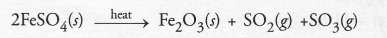The gases evolved are a mixture of sulphur dioxide and sulphur trioxide. Both have the smell of burning sulphur.

Question 28.
Why do fire flies glow at night ?
Fire flies are associated with certain protein. In the presence of a enzyme, the protein undergoes aerial oxidation. The reaction is accompanied by emission of light. As a result, fire flies glow at night.

Question 29.
Grapes hanging on the plants do not ferment but after being plucked from the plant, can be fermented. Under what conditions do these grapes ferment ? Is it a chemical or a physical change ?
Grapes hanging on the plants are living in nature. Their immune system does not allow any fermentation.
However, when these are plucked, they are no longer living. They undergo fermentation under aerobic conditions (in the absence of air or oxygen). The other factors which favour fermentation are enzyme and temperature between 25°C to 30°C. The fermentation is a chemical change.

Question 30.
Which among the following are physical or chemical changes ?
(a) Evaporation of petrol
(b) Burning of Liquefied Petroleum Gas (LPG)
(c) Heating of an iron rod to red hot.
(d) Curding of milk
(e) Sublimation of solid ammonium chloride.
(a) Physical
(b) Chemical
(c) Physical
(d) Chemical
(e) Physical.

Question 31.
During the reaction of some metals with dilute hydrochloric acid, following observations are made.
(a) Silver metal does not show any change
(b) The temperature of the reaction mixture rises when aluminium (Al) is added.
(c) The reaction of sodium metal is found to be highly explosive
(d) Some bubbles of a gas are seen when lead (Pb) is reacted with the acid.
Explain these observations giving suitable reasons.
(a) Silver (Ag) is placed below hydrogen in the activity series. It cannot replace hydrogen from dilute HCl and no chemical reaction is possible.
(b) The reaction of aluminium (Al) with dilute HCl is of exothermic nature. Therefore the temperature rises.
(c) Hydrogen gas is evolved when sodium metal (Na) is reacted with dilute HCl. The reaction is of exothermic nature. Therefore, hydrogen catches fire accompanied by explosion.
2Na (s) + 2HCl(aq) ———-> 2NaCl(aq) + H2(g)
Remember : Sodium metal as such does not catch fire. It is the hydrogen gas evolved which burns since the reaction is highly exothermic in nature.
(d) Lead (Pb) reacts with dilute HCl to evolve hydrogen gas accompanied by bubbles.
Pb(s) + 2HCl (aq) ———-> PbCl2(aq) + H2(g).

Question 32.
A substance ‘A’, which is an oxide of a group 2 element, is used intensively in the cement industry. This element is present in bones also. On treatment with water it forms a solution which turns red litmus blue. Identify A’ and also write the chemical reactions involved.
The available information suggests that the substance A’ is oxide of the element calcium (Ca) which is present in group 2 of the periodic table. Calcium is also a constituent of our bones in the form of calcium phosphate. . Calcium oxide (CaO) reacts with water to form calcium hydroxide (basic in nature). It forms a basic solution which turns red litmus blue.Question 33.
Write a balanced chemical equation for each of the following reactions and also classify them.
(a) Lead acetate solution is treated with dilute hydrochloric acid to form lead chloride and acetic acid solution.
(b) A piece of sodium metal is added to absolute ethanol to form sodium ethoxide and hydrogen gas.
(c) Iron (III) oxide on heating with carbon monoxide gas reacts to form solid iron and liberates carbon dioxide gas.
(d) Hydrogen sulphide gas reacts with oxygen gas to form solid sulphur and liquid water. (CBSE 2014)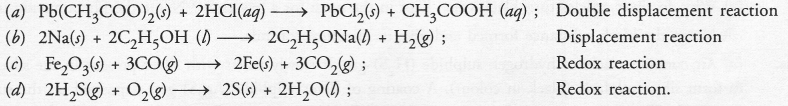Question 34.
Why do we store silver chloride in a dark coloured bottle ?
Silver chloride is a white solid. However, it is very senstive to sun light and decomposes in the presence of light to form silver (grey in colour) and chlorine.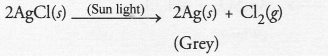In order to avoid action by sun light, it is kept in a coloured bottle.

Question 35.
Balance the following chemical equations and identify the type of chemical reaction.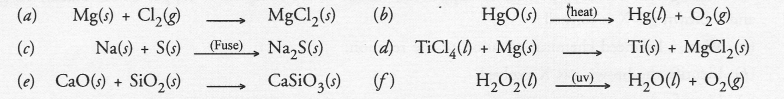The chemical equations in their balanced form may be written as follows :Question 36.
A magnesium ribbon is burnt in oxygen to give a white compound ‘X’ accompanied by emission of light. If the burning ribbon is now placed in an atmosphere of nitrogen, it continues to burn and forms a compound ‘Y’.
(a) Write the chemical formulae of X and Y.
(b) Write a balanced chemical equations when X and Y are dissolved in water.
(a) The Compound ‘X’ is magnesium oxide (MgO). The compound ‘Y’ is magnesium nitride (Mg3N2)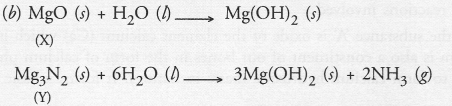Question 37.
Zinc liberates hydrogen gas when reacted with dilute hydrochloric acid whereas copper does not. Explain why ?
Zinc (Zn) is placed above hydrogen in the activity series. It therefore, displaces hydrogen from dilute HCl.
Zn (s) + 2HCl (aq) ————-> ZnCl2 (aq) + H2(g)
Copper is placed below hydrogen and therefore, does not react with dilute HCl.
Cu (s) + HCl (aq) ———–> No action.

Question 38.
A silver article generally turns black when kept in the open for a few days. The article when rubbed with toothpaste again starts shining.
(a) Why do silver articles turn black when kept in the open for a few days ? Name the phenomenon involved.
(b) Name the black substance formed and give its chemical formula.
(a) Air contains traces of hydrogen sulphide (H2S) gas. It slowly reacts with silver present in the silver article to form silver sulphide (black in colour). A coating of silver sulphide (Ag2S) gets deposited on the surface of the metal. The phenomenon is known as corrosion. Tooth pastes generally contain traces of hydrogen peroxide as one of the constitutents. It reacts with silver sulphide to form silver sulphate which is white in colour. Therefore, whiteness is restored to the article.
(b)NCERT Exemplar Solutions for Class 10 Science Chapter 1 Long Answer Questions

Question 39.
On heating blue coloured powder of copper (II) nitrate, in a boiling tube, copper oxide (black), oxygen gas and a brown gas X is formed
(a) Write a balanced chemical equation of the reaction.
(b) Identify the brown gas X evolved.
(c) Identify the type of reaction.
(d) What could be the pH range of aqueous solution of the gas X ?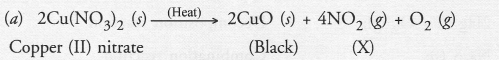(b) Brown gas (X) is nitrogen dioxide.
(c) It is an example of decomposition reaction.
(d) Nitrogen dioxide is an acidic oxide. Therefore, it dissolves in water to form an acidic solution. The pH of the solution is expected to be less than 7.

Question 40.
Give the characteristic tests for the following gases :
(a) CO2
(b) SO2
(c) O2
(d) H2
(a) CO2 gas : It is a colourless and odourless gas. When passed through lime water, it will initially become milky.(b) SO2 gas : It is a colourless but a pungent smelling gas. The gas when passed through an acidified solution of potassium permanganate (pink) will decolourise it.Similarly the gas when passed through an acidified solution of potassium dichromate (orange) will make it light green.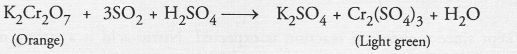(c) Oxygen gas : The gas is a supporter of combustion. It can be tested by bringing a burning or smouldering match-stick near the mouth of the test tube from which the gas escapes. The match-stick will burn more brightly.
(d) Hydrogen gas : The gas itself is combustible. It will burn with a pop sound when a burning candle or match- stick is brought near it.
2H2 (g) + O2 (g) ———-> 2H2O (g)

Question 41.
What happens when a piece of
(a) zinc metal is added to copper sulphate solution ?
(b) aluminium metal is added to dilute hydrochloric acid ?
(c) silver metal is added to copper sulphate solution ?
Also write the balanced chemical equation if the reaction occurs.
(a) Metal zinc displaces hydrogen component on reacting with dilute sulphuric acid (H2SO4) as H2 gas which is evolved
Zn(s) + H2SO2(aq) ———-> ZnSO4(aq) + H2(g)
(b) Ammonia and hydrogen chloride gas. When vapours of ammonia and hydrogen chloride gas are mixed, the compound formed is ammonium chloride. It is a white solid.
NH3(g) + HCl(g) ———-> NH4Cl (s)
(c) Copper displaces silver from the aqueous solution of silver nitrate.
Cu(s) + 2AgNO3(aq) ——–> Cu(NO3)2(aq) + 2Ag(s)

Question 42.
What happens when zinc granules are treated with dilute solution of H2SO4, HCl, HNO3, NaCl and NaOH ? Also write the chemical equations if reaction oçcurs.
With dilute H2SO4 : Hydrogen gas evolves
Zn (s) + H2SO4 (dil.) ————> ZnSO4 (aq) + H2(g)
with dilute HCl : Hydrogen gas evolves
Zn (s) + 2HCl (dil.) ———–> ZnCl2(aq) + H2(g)
with dilute HNO3 : Nitrous oxide gas evolves. It is colourless.
4Zn (s) + 10HNO3 (aq) ———->  4Zn(NO3)2 (aq) + 5H2O (l) + N2O(g)
with NaCl : There is no chemical reaction, with NaOH : Hydrogen gas evolves :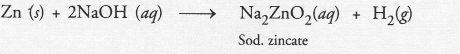Question 43.
On adding a drop of barium chloride solution to an aqueous solution of sodium sulphite, white precipitate is obtained :
(a) Write a balanced chemical equation for the reaction involved.
(b) What other name can be given to this precipitation reaction ?
(c) On adding dilute hydrochloric acid to the reaction mixture, white precipitate disappears. Why ?(b) The precipitation reaction is also called double displacement reaction.
(c) White precipitate of barium sulphite reacts with dilute hydrochloric to form barium chloride and sulphur dioxide gas. Since barium chloride is water soluble, the white precipitate will slowly disappear.Question 44.
You are provided with two containers made up of copper and aluminium. You are also provided with solutions of dilute HCl, dilute HNO3, ZnCl2 and water. In which of the above containers, can these solutions be kept ?
Solutions to be kept in copper container :

1. Dilute HCl can be kept since no chemical reaction is expected. Copper is placed below hydrogen in the activity series.
2. Dilute HNO3 cannot be kept since a chemical reaction is expected. Nitric acid is a strong oxidising agent.
3Cu (s) + 8HNO3 (dit) ———–> 3 CU(NO3)2 (aq) + 2NO (g) + 4H2O (aq)
3. ZnCl2 solution can be kept since copper is placed below zinc in the activity series and no chemical reaction is possible.
4. Water can be kept since no chemical reaction is expected.

Solutions to be kept in aluminium container:

1. Dilute HCl cannot be kept since aluminium reacts with acid to evolve hydrogen gas.
2Al (s) + 6HCl (aq) ———-> 2AlCl3 (aq) + 3H2 (g)
2. Dilute HNO3 can be kept. Actually, aluminium is initially oxidised by the acid to aluminium oxide (Al2O3). A coating of aluminium oxide gets deposited on the surface of the metal and makes it passive towards reaction with the acid.
3. ZnCl2 solution cannot be kept since a chemical reaction will take place as aluminium is placed above zinc in the activity series.
2Al (s) + 3ZnCl2 (aq) ———-> 2AlCl3 (aq) + 3Zn (s)
4. Water as such can be kept in the container. However, we cannot keep steam since a chemical reaction is likely to take place.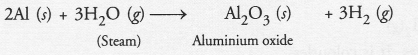Hope given NCERT Exemplar Solutions for Class 10 Science Chapter 1 Chemical Reactions and Equations are helpful to complete your science homework.

If you have any doubts, please comment below. Learn Insta try to provide online science tutoring for you.